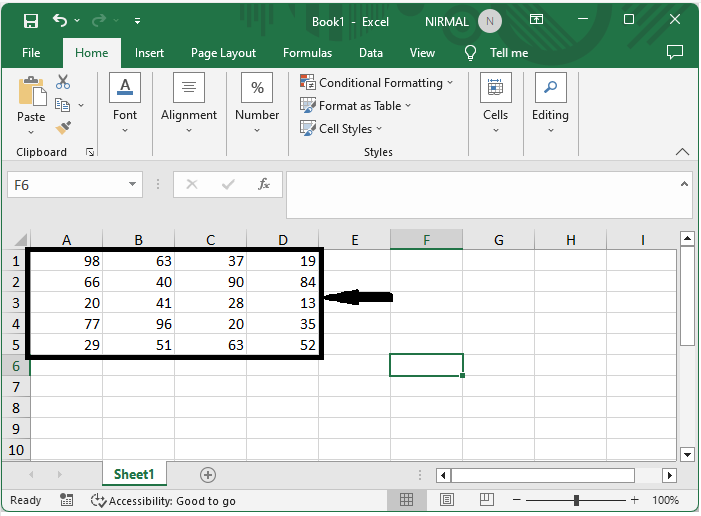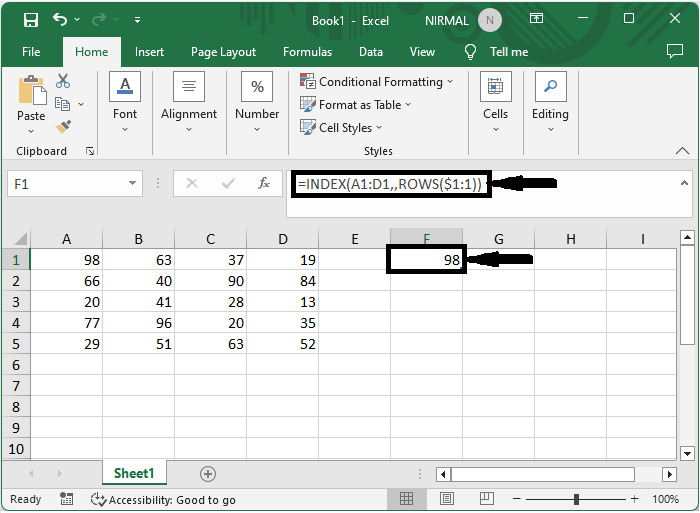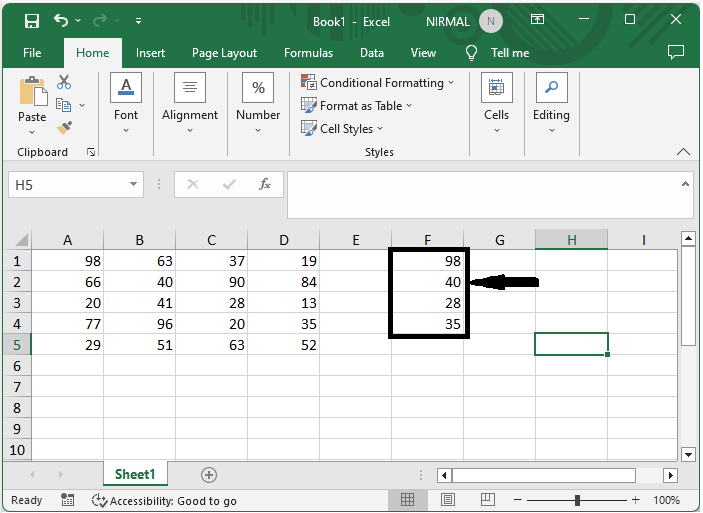# How to Extract Diagonal Matrix in Excel

You've come to the right place if you're using Excel to work with matrices and need to extract only the diagonal elements. A matrix's diagonal elements are those that are located on the major diagonal, which runs from top left to bottom right. When performing calculations or data analysis, extracting these pieces might be a valuable activity. In this article, we'll show you how to use Excel's built−in functions and formulas to extract the diagonal members of a matrix. This tutorial will give you step−by−step instructions and examples to help you comprehend and put the extraction process into practise, regardless of your level of Excel proficiency.

Make sure you have a basic understanding of Excel and are comfortable using matrices and calculations before we start. Don't worry if you're new to Excel. We'll lay out the stages succinctly and clearly so you can follow along. You will have the knowledge and abilities necessary to extract the diagonal matrix elements in Excel by the end of this tutorial, which will enable you to complete your data analysis jobs faster and with less effort. In order to extract the diagonal matrix in Excel, let's get started.

## Extracting a Diagonal Matrix in Excel

Here we will first use a formula to get any one of the results, then use the autofill handle to complete the task. So let us see a simple process to know how you can extract a diagonal matrix in Excel.

Step 1 : Consider an Excel sheet where you have data in matrix format, similar to the below image.First, click on an empty cell and enter the formula as =INDEX(A1:D1,,ROWS(\$1:1)) and click enter to get the first value.

Empty cell > Formula > Enter.Step 2 :Then drag down using the auto−fill handle till you fill all the values. Then delete the error values to complete the task.## Conclusion

In this tutorial, we have used a simple example to demonstrate how you can extract a diagonal matrix in Excel to highlight a particular set of data.

Updated on: 20-Jul-2023

699 Views